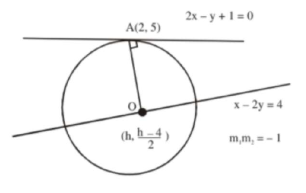# The lineQuestion:

The line $2 \mathrm{x}-\mathrm{y}+1=0$ is a tangent to the circle at the point $(2,5)$ and the centre of the circle lies on $x-2 y=4$. Then, the radius of the circle is:

1. (1) $3 \sqrt{5}$

2. (2) $5 \sqrt{3}$

3. (3) $5 \sqrt{4}$

4. (4) $4 \sqrt{5}$

Correct Option: 1

Solution:$\left.\begin{array}{l}\left(\frac{\mathrm{h}-\frac{(\mathrm{h}-\mathrm{i})}{2}}{2-\mathrm{h}}\right)(2)--1 \\ \mathrm{~h}=8\end{array}\right)$

center $(8,2)$

Radius $\left.=\sqrt{(8-2)^{2}+(2-5)^{2}}=3 \sqrt{5}\right)$